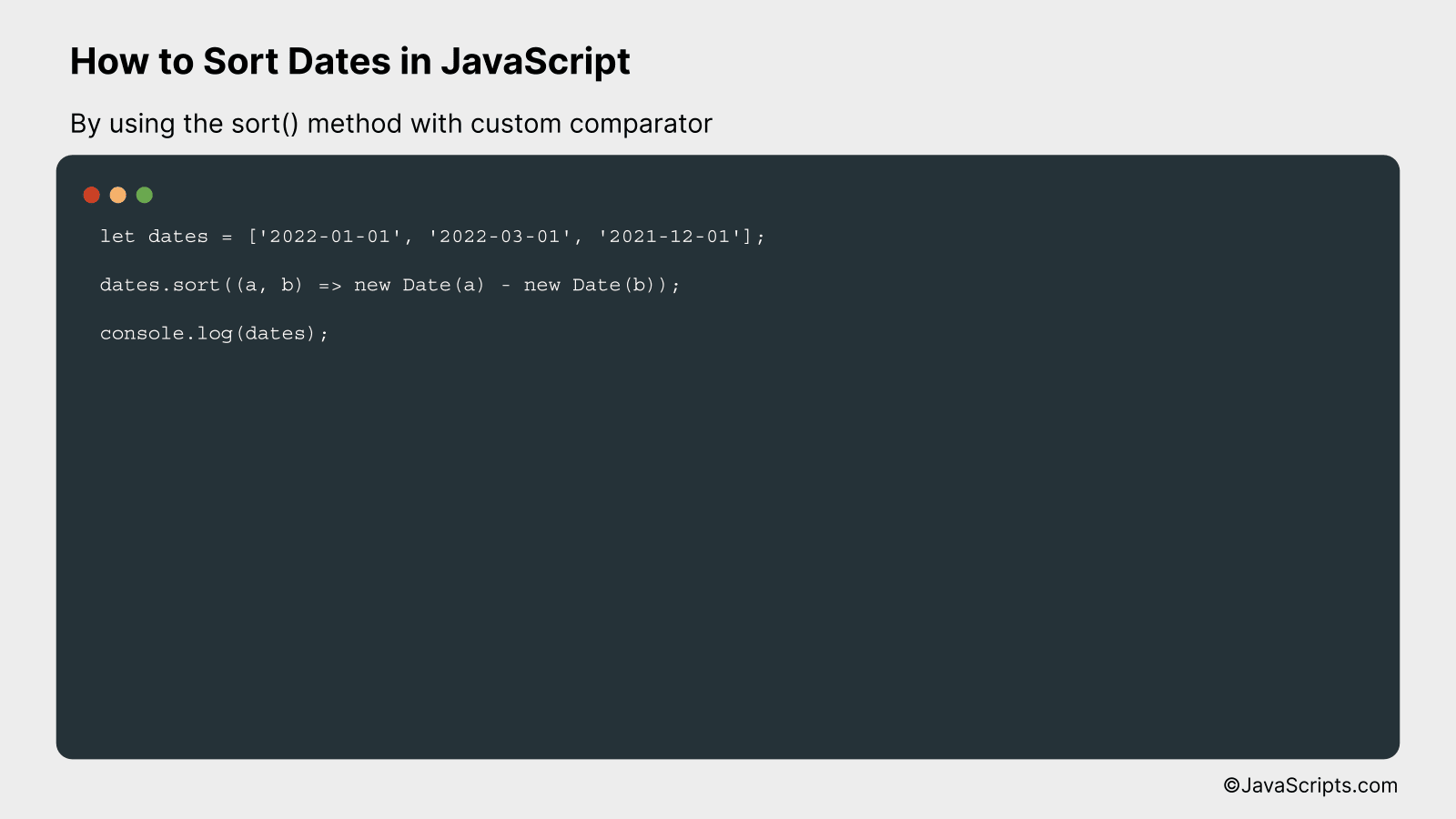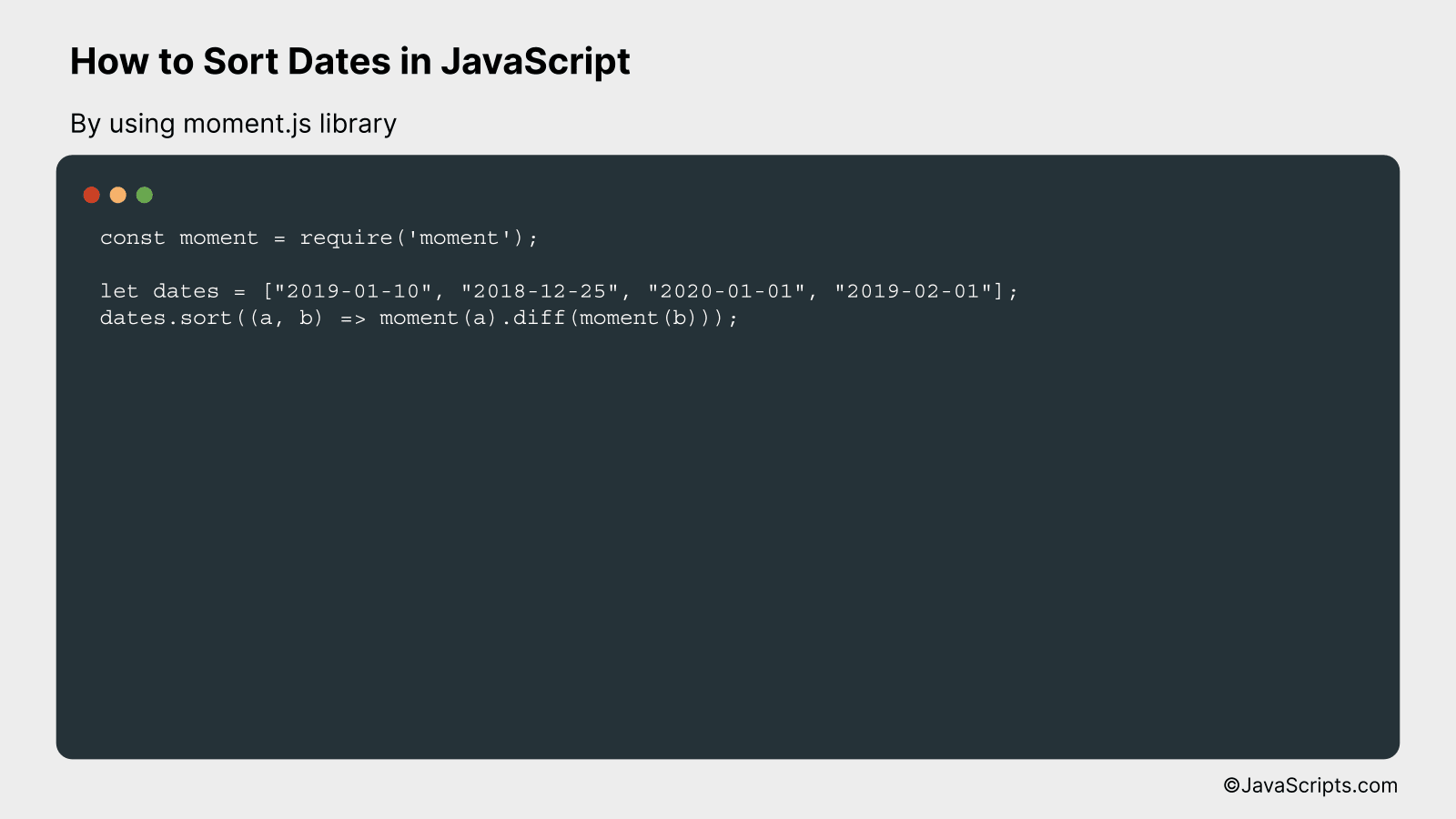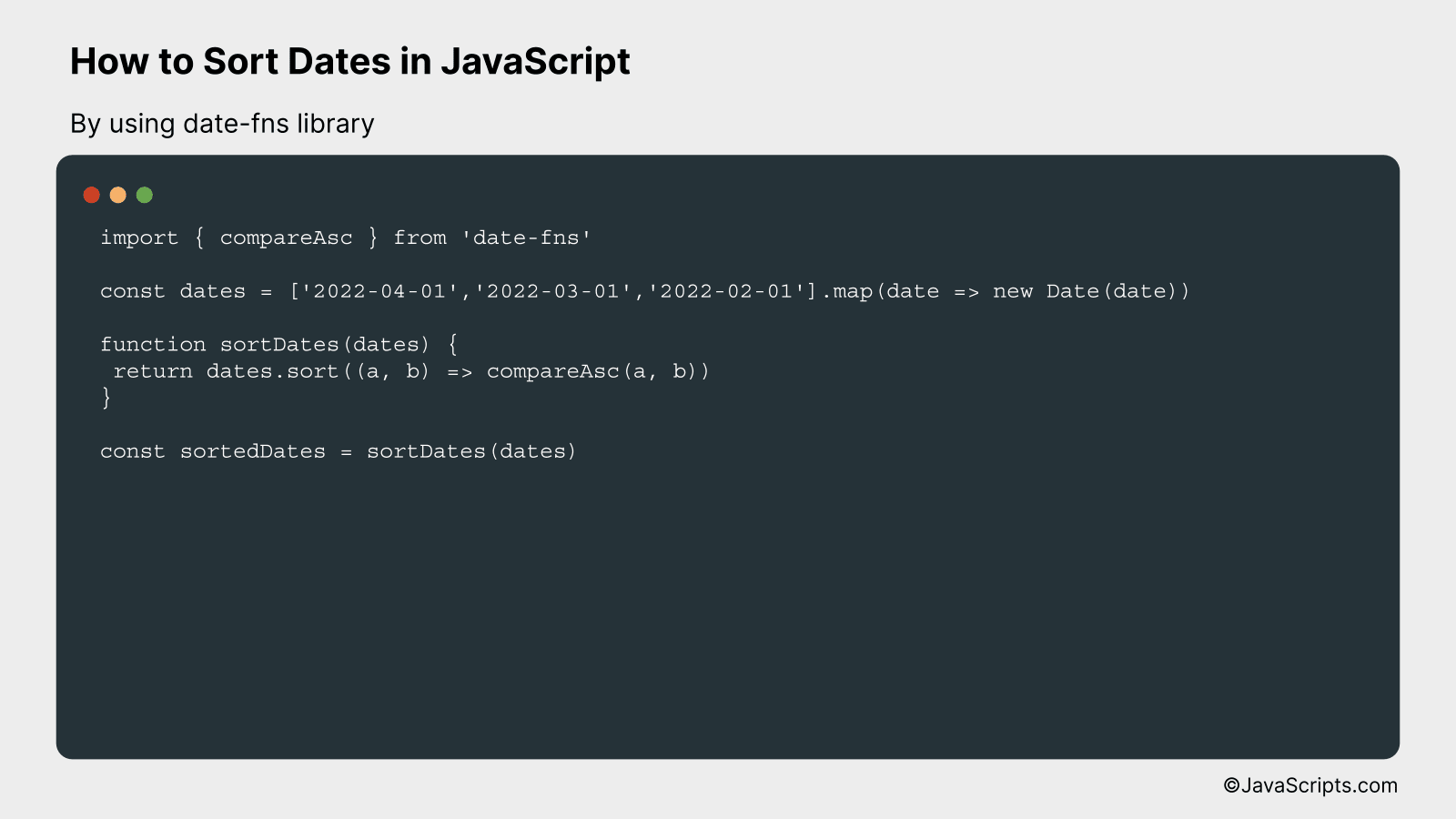# How to Sort Dates in JavaScript

JavaScript, the backbone of modern web development, is an essential skill in today’s digital world. While it’s an incredibly versatile language, handling dates can be a little tricky even for seasoned developers. That’s where mastering the art of manipulating and sorting dates in JavaScript becomes crucial.

Imagine you’re building a blog website where posts need to be displayed in chronological order, or a calendar app that sorts events based on their dates. In such scenarios, would you know how to go on about sorting dates? Don’t worry, because together we’ll walk through some simple and effective strategies to conquer this challenge.

In this post, we will be looking at the following 3 ways to sort dates in JavaScript:

• By using the sort() method with custom comparator
• By using moment.js library
• By using date-fns library

Let’s explore each…

## #1 – By using the sort() method with custom comparatorWe are going to sort an array of dates in JavaScript by using the sort() method with a custom comparator. The comparator function will take two dates and compare them, rearranging their order based on which is earlier. This will be demonstrated with a simple array of date strings.

``````
let dates = ['2022-01-01', '2022-03-01', '2021-12-01'];
dates.sort((a, b) => new Date(a) - new Date(b));
console.log(dates);
``````

### How it works

This code works by providing a custom sort function to the JavaScript array sort() method. This function takes two dates as input and subtracts one from the other to determine the sort order. As a result, the date array is sorted in ascending order.

• The code declares a dates array containing different date strings.
• The sort() method is called on the dates array with a custom comparator function as its argument.
• The comparator function takes two arguments, a and b. These represent any two elements in the array.
• Inside the comparator function, a and b are converted into Date objects and subtracted from one another. This results in a numeric value that indicates the time difference between the two dates.
• If the result is less than 0, a is sorted to an index lower than b (i.e., a comes first).
• If the result is 0, the order of a and b is unchanged with respect to each other.
• If the result is more than 0, a is sorted to an index higher than b (i.e., b comes first).
• Finally, the sorted dates array is printed to the console, in ascending chronological order.

## #2 – By using moment.js libraryWe will be sorting an array of dates in JavaScript using the Moment.js library. This procedure works by converting each string date into a Moment.js date object, which we can then sort in ascending order. Here’s an example:

``````
const moment = require('moment');

let dates = ["2019-01-10", "2018-12-25", "2020-01-01", "2019-02-01"];
dates.sort((a, b) => moment(a).diff(moment(b)));
```
```

### How it works

The code uses the Moment.js library to convert strings to date objects, which can be correctly sorted in JavaScript. The sorting is done by using the Array.sort() method along with Moment’s diff() function.

• First, we create an array of dates in string format. These dates are unsorted.
• Next, we use the sort() method provided by JavaScript’s Array prototype. This method sorts the elements of an array in place and returns the array.
• The sort() method accepts a compare function, which dictates the sort order.
• We provide a compare function that takes two dates, converts them to Moment.js date objects, and then uses the diff() function to find the difference between the two dates.
• The diff() function returns a positive number if the first date is after the second, a negative number if the first date is before the second, and 0 if the dates are equal. This fits the requirements of the compare function for sort().
• The sorted array will be in ascending order, i.e., from the earliest to the latest date.

## #3 – By using date-fns libraryThe following approach will demonstrate how to sort an array of dates in JavaScript using the ‘date-fns’ library, by comparing the dates in a sort function. A code snippet followed by a detailed explanation will make the concept clear.

``````
import { compareAsc } from 'date-fns'

const dates = ['2022-04-01','2022-03-01','2022-02-01'].map(date => new Date(date))

function sortDates(dates) {
return dates.sort((a, b) => compareAsc(a, b))
}

const sortedDates = sortDates(dates)
``````

### How it works

The code first imports the ‘compareAsc’ function from ‘date-fns’ library, which is used to compare two dates. It then uses this function inside the JavaScript array ‘sort’ function to sort the array of dates in ascending order.

• First, the ‘compareAsc’ function is imported from the ‘date-fns’ library. This function compares two dates and returns a number indicating whether one date is before, equal, or after the other.
• Then an array of date strings is created and each string is converted into a Date object with the ‘map’ function.
• A function named ‘sortDates’ is defined that takes an array of dates as an argument. Inside this function, the ‘sort’ method is called on the array of dates. The ‘sort’ method takes a comparator function as an argument, which in this case is provided by ‘compareAsc’.
• The ‘compareAsc’ function compares two dates at a time, and the ‘sort’ method uses this comparison to arrange the dates in ascending order. If ‘compareAsc’ returns a value less than 0, date ‘a’ is sorted to be earlier than date ‘b’. If it returns a value greater than 0, ‘a’ is sorted to be later than ‘b’. If it returns 0, ‘a’ and ‘b’ are considered equal.
• Finally, the sorted array of dates is stored in the ‘sortedDates’ variable.

Related:

In conclusion, sorting dates in JavaScript is not as daunting as it may first appear. With the use of inbuilt JavaScript Date objects and array sort function, we can easily sort dates in an array.

Remember, the key is to transform our date strings into dates that JavaScript can understand, allowing us to sort them correctly. With practice, this process will become second nature.